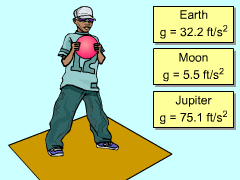Ch 1. Particle General Motion Multimedia Engineering Dynamics Position,Vel & Accel. Accel. varyw/ Time Accel. Constant Rect. Coordinates Norm/Tang. Coordinates Polar Coordinates RelativeMotion
 Chapter - Particle - 1. General Motion 2. Force & Accel. 3. Energy 4. Momentum - Rigid Body - 5. General Motion 6. Force & Accel. 7. Energy 8. Momentum 9. 3-D Motion 10. Vibrations Appendix Basic Math Units Basic Equations Sections Search eBooks Dynamics Fluids Math Mechanics Statics Thermodynamics Author(s): Kurt Gramoll ©Kurt GramollDYNAMICS - CASE STUDY IntroductionGravity on a Falling Ball on Different Planets A boy trying to understand gravity holds a ball above the earth and lets it fall. With aspirations of being a rocket scientist, he wonders how fast the ball would fall on another planetary bodies. What is known: The ball is released from rest 4 ft above the earth. The gravitational constant is 32.2 ft/s2 towards the earth. Questions What is the velocity of the ball when it hits the earth? If the ball is being released from rest and is to have a speed of 14 mph when it hits the earth, from what height should it be dropped? Approach With the acceleration given as a constant, the velocity and position equations can be found by integrating the relationships, a = dv/dt and v = dx/dt.

Practice Homework and Test problems now available in the 'Eng Dynamics' mobile app
Includes over 400 problems with complete detailed solutions.
Available now at the Google Play Store and Apple App Store.